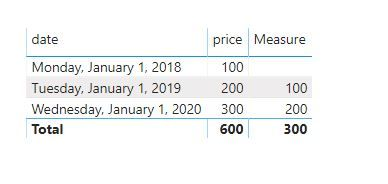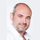cancel
Showing results for
Did you mean:Anonymous
Not applicable

## Put data from y to y-1 based on costumer selection

Hello Folks,

Happy new year, i need your help about a tricky subject. My client want's to analyse 2019 data and compare it with 2018. I trying to use DATEADD ou PREVIOUSPERIOD but those formulas based their calculation on system date. so  y = 2020 and y-1 is 2019.

What formulas can i use for display  "price y-1"  with dynamic date base ? See my sample below

 date price date y-1 price y-1 01/01/2018 100 01/01/2017 01/01/2019 200 01/01/2018 100 01/01/2020 300 01/01/2019 200

Thanks a lot 🙂

Regards,

1 ACCEPTED SOLUTIONCommunity Support

hi  @Anonymous

You could use DATEADD Function to get it.here is sample pbix file, Please try it.

Regards,

Lin

Community Support Team _ Lin
If this post helps, then please consider Accept it as the solution to help the other members find it more quickly.
4 REPLIES 4Community Support

hi  @Anonymous

You could use DATEADD Function to get it.here is sample pbix file, Please try it.

Regards,

Lin

Community Support Team _ Lin
If this post helps, then please consider Accept it as the solution to help the other members find it more quickly.Anonymous
Not applicable

Thanks a lot guys that works ! 🙂Super User

Make sure you have a date calendar defined.

To get the best of the time intelligence function. Make sure you have a date calendar and it has been marked as the date in model view. Also, join it with the date column of your fact/s.

Refer

After that try

``````12 Month behind = CALCULATE(SUM(Sales[Sales Amount]),dateadd(('Date'[Date]),-1,Year))

YTD Sales = CALCULATE(SUM(Sales[Sales Amount]),DATESYTD(('Date'[Date])))

Last YTD Sales = CALCULATE(SUM(Sales[Sales Amount]),DATESYTD(dateadd('Date'[Date],-1,Year)))
Last YTD Sales = CALCULATE(SUM(Sales[Sales Amount]),DATESYTD(ENDOFYEAR(dateadd('Date'[Date],-1,Year))))

MTD Sales = CALCULATE(SUM(Sales[Sales Amount]),DATESMTD('Date'[Date]))
last MTD Sales = CALCULATE(SUM(Sales[Sales Amount]),DATESMTD(dateadd('Date'[Date],-1,MONTH)))
last MTD (complete) Sales =  CALCULATE(SUM(Sales[Sales Amount]),DATESMTD(ENDOFMONTH(dateadd('Date'[Date],-1,MONTH))))
last year MTD (complete) Sales =  CALCULATE(SUM(Sales[Sales Amount]),DATESMTD(ENDOFMONTH(dateadd('Date'[Date],-12,MONTH))))

MTD (Year End) Sales =  CALCULATE(SUM(Sales[Sales Amount]),DATESMTD(ENDOFYEAR('Date'[Date])))
MTD (Last Year End) Sales =  CALCULATE(SUM(Sales[Sales Amount]),DATESMTD(ENDOFYEAR(dateadd('Date'[Date],-12,MONTH),"8/31")))

QTD Sales = CALCULATE(SUM(Sales[Sales Amount]),DATESQTD(('Date'[Date])))

Last QTD Sales = CALCULATE(SUM(Sales[Sales Amount]),DATESQTD(dateadd('Date'[Date],-1,QUARTER)))
Next QTD Sales = CALCULATE(SUM(Sales[Sales Amount]),DATESQTD(dateadd('Date'[Date],1,QUARTER)))

Last year same QTD Sales = CALCULATE(SUM(Sales[Sales Amount]),DATESQTD(dateadd('Date'[Date],-1,Year)))``````

Appreciate your Kudos. In case, this is the solution you are looking for, mark it as the Solution. In case it does not help, please provide additional information and mark me with @
Thanks. My Recent Blog -
https://community.powerbi.com/t5/Community-Blog/Winner-Topper-on-Map-How-to-Color-States-on-a-Map-wi...
https://community.powerbi.com/t5/Community-Blog/HR-Analytics-Active-Employee-Hire-and-Termination-tr...
https://community.powerbi.com/t5/Community-Blog/Power-BI-Working-with-Non-Standard-Time-Periods/ba-p...
https://community.powerbi.com/t5/Community-Blog/Comparing-Data-Across-Date-Ranges/ba-p/823601Frequent Visitor

Hi,

You're right DATEADD() is correct, and to make it work you have to use it inside CALCULATE()

Price LY =

CALCULATE (

SUM ( [price] ),

DATEADD ( [Date], -1 , YEAR )

)

Should work 😉 let us know